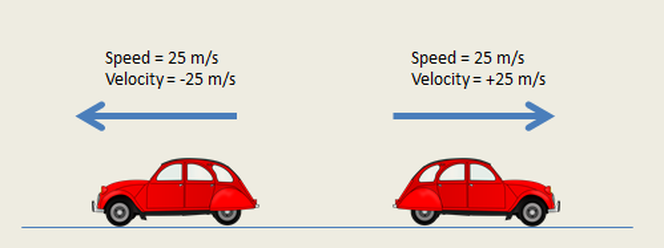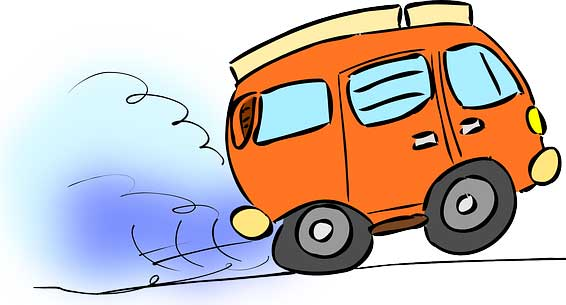# Motion Study Guide

INTRODUCTION

How often have you stopped to consider what motion really is? You are on your way to school on the bus, and your football is in your seat. The bus is moving at twenty miles an hour relative to the ground, but so is your football! Is the ball at rest, or is it in motion? 🤔

Consider all the things around you; although they look as if they are at rest, they are still spinning around the earth’s axis and going around the sun. How would you describe all this motion? Not everything moves in the same way or at the same speed, so let’s find out how!Source

### WHAT IS MOTION?

The definition of motion in physics, very simply put, is the change in position of an object relative to a frame of reference, such as its surroundings during a defined interval of time. Motion is described using several factors such as distance, displacement, time, speed, velocity, and acceleration. If you are sitting on a bench in a park, you are said to be at rest with respect to the park. When you get up to leave, then you are said to be in motion. In science, the basic attributes of motion are commonly measured using velocity and acceleration.

### WHAT IS VELOCITY?Source

We are all familiar with the concept of speed – it gives a measure of how fast we are travelling. However, speed doesn’t really indicate the direction of motion since speed is a scalar concept (one-dimensional) and tells us only the rate of change of distance with time.

Velocity is the rate of change of displacement with time. Velocity is a more useful concept in physics as it specifies a direction in addition to speed, making it a vector quantity (a vector quantity indicates both magnitude and direction). It is important to remember that since it indicates direction, velocity can also be negative!

Mathematically the average velocity can be represented as:

``                     v = Δx/Δt``
• v is the average velocity
• Δx is the change in displacement
• t is the elapsed time

The unit of velocity is meters per second, similar to spee (m/s).

### WHAT IS ACCELERATION?Source

Understanding the concept of motion is not complete without the concept of acceleration. We just went over the concept of velocity, which is the rate of change of displacement.

Acceleration, in turn, is the rate of change of velocity. When you go down a sloping road on your scooter, you tend to go faster with every passing second, and that is basically what acceleration is! Your velocity continues to increase as you accelerate down the slope. Mathematically acceleration is represented as:

``                          a = Δv/Δt``

a is the acceleration Δv is the change in velocityΔt is the change in time

The unit of acceleration is m/s2.

## CONCLUSION:

• Motion is described using quantities such as speed, velocity, and acceleration.
• Velocity is the rate of change of displacement.
• Acceleration is the rate of change of velocity.

### FAQs:

1. What do we mean by motion?

The motion definition in Physics talks about the change in position of an object with respect to a frame of reference over time.

2. What are the 4 types of motion?

The 4 types of motion are:

• Translational motion
• Rotational motion
• Oscillatory motion
• Random motion

3. What are examples of motion?

Motion in science relates to the change in position of an object with respect to a frame of reference over time. Examples include the motion of cars and vehicles on the street, the flight of birds, flowing water, etc.

We hope you enjoyed studying this lesson and learned something cool about Motion! Join our Discord community to get any questions you may have answered and to engage with other students just like you! We promise, it makes studying much more fun! 😎

## SOURCES:

1. Motion. https://flexbooks.ck12.org/studyguides/physics/motion-study-guide.html?encodedID=SCI.PHY.152&courseContextID=4283481. Accessed 27 Jan 2022.
2. Motion in Physics. https://byjus.com/physics/motion-in-physics/. Accessed 27 Jan 2022.
3. What is velocity?. https://www.khanacademy.org/science/physics/one-dimensional-motion/displacement-velocity-time/a/what-is-velocity. Accessed 27 Jan 2022.
]]>